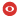Statut Confirmé Série IPN-X Domaines hep-ph Date Jeudi 8 Novembre 2018 Heure 11:00 Institut CPHT Salle Salle de Conferences Louis Michel, bat. 6, CPHT, Ecole polytechnique Nom de l'orateur Pham Prenom de l'orateur Tri Nang Addresse email de l'orateur Institution de l'orateur CPHT, Ecole polytechnique Titre Heavy to Light Meson Semileptonic Decays Form Factors Résumé Like the two-photon and two-gluon decays of the $P$-wave $\chi_{c0,2}$ and $\chi_{b0,2}$ state for which the Born term produces a very simple decays amplitude in terms of an effective Lagrangian with two-quark local operator, the Born term for the processes $c\bar{d}\to(\pi,K)\ell\nu$ and $b \bar{d}\to(\pi, K)\ell\nu$, could also produce the $D$ and $B$ meson semileptonic decays with the light meson $\pi, K$ in the final state. In this approach to heavy-light meson form factors with the $\pi, K$ meson treated as Goldstone boson, a simple expression is found for the decays form factors, given as~: $f_{+}(0)/(1-q^{2}/(m_{H}^{2}+m_{\pi}^{2}))$, with $H=D,B$ for $D,B\to\pi$ form factors, and $f_{+}(0)/(1-q^{2}/(m_{H}^{2}+ m_{K}^{2}))$ for $B, D\to K$ form factor. In this talk, I would like to present a recent work showing that this expression for the form factors could describe rather well the $q^{2}$-behaviour observed in the BaBar, Belle and BESSIII measurements and lattice simulation. In particular, the $D\to K$ form factors are in good agreement with the measured values in the whole range of $q^{2}$ showing evidence for $SU(3)$ breaking with the presence of $m_{K}^{2}$ term in the quark propagator, but some corrections to the Born term are needed at large $q^{2}$ for $D, B\to\pi$ form factors. Numéro de preprint arXiv Commentaires Fichiers attachés 2018-11-08_slides.pdf (99443 bytes)Pour obtenir l' affiche de ce séminaire : [ Postscript | PDF ]

 [ Annonces ]    [ Abonnements ]    [ Archive ]    [ Aide ]    [ JavaScript requis ] [ English version ]